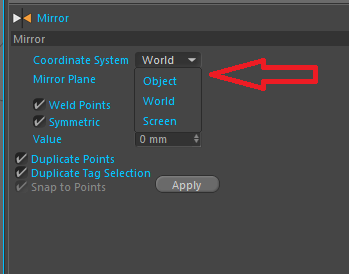# Setting `coordinate system` using `MCOMMAND_MIRROR`

• Hi there, I'm trying to mirror object via `SendModelingCommand(c4d.MCOMMAND_MIRROR, ..)`
But I cann't understand how to change a coordinate system.
According to the documentation this options is called `MDATA_MIRROR_SYSTEM` and it's `int` type.
Its description says `Coordinate system. (See dialog.)`
I believe it is the dialog:It seems like 0 means Object, 1 - World, 2 - Screen.
I tried different values from 0 to 30, but it didn't take any effect.

The code I'm using:

``````settings = c4d.BaseContainer()
settings[c4d.MDATA_MIRROR_SYSTEM] = 0

res = utils.SendModelingCommand(c4d.MCOMMAND_MIRROR,
[obj],
c4d.MODELINGCOMMANDMODE_ALL,
settings,
doc)
``````

I cannot change `MDATA_MIRROR_PLANE` either.
There is no additional information in the documentation.
Drag'n'drop for these fields doesn't work. It's strange, because it works perfectly for other tools.

I found this post (from 2011) https://plugincafe.maxon.net/topic/5497/5516_mirror-tool-bug
It seems that bug is still alive :(

Version: R20
Platform: Windows

Cheers

• Hi @kisaf,

MCOMMAND_MIRROR is indeed a bit special because when you click on the Apply button from the GUI, it does send a message to some c4d elements, then these elements read MDATA_MIRROR_VALUE, MDATA_MIRROR_SYSTEM and MDATA_MIRROR_PLANE calculates values for MDATA_MIRROR_POINT and MDATA_MIRROR_VECTOR and then call the SendModelingCommand. Unfortunately, there is no way for you to send this message, and the best way to do it is to recalculate the stuff as bellow.

``````import c4d

def main():
# Checks if selected object is valid
if op is None:
raise ValueError("op is none, please select one object.")

# Defines some general settings, take care MDATA_MIRROR_VALUE, MDATA_MIRROR_SYSTEM and MDATA_MIRROR_PLANE
settings = c4d.BaseContainer()
settings[c4d.MDATA_MIRROR_DUPLICATE] = True
settings[c4d.MDATA_MIRROR_SELECTIONS] = True
settings[c4d.MDATA_MIRROR_ONPLANE] = True
settings[c4d.MDATA_MIRROR_WELD] = True
settings[c4d.MDATA_MIRROR_TOLERANCE] = 0.01

# Corresponds to MDATA_MIRROR_VALUE, MDATA_MIRROR_SYSTEM and MDATA_MIRROR_PLANE
value = 1000.0
system = 0 # 0 = Object, 1 = World
plane = 1 # 0 = XY, 1 = ZY, 2 = XZ

if not 0 <= system < 2:
raise ValueError("System can only be 0 or 1")

# Buffer position vector
pos = c4d.Vector()

# Calculates Local (Object) coordinates
if system == 0:
globalMatrix = op.GetMg()
if plane == 0:
pos = globalMatrix.v3
elif plane == 1:
pos = globalMatrix.v1
elif plane == 2:
pos = globalMatrix.v2

settings[c4d.MDATA_MIRROR_POINT] = globalMatrix.off + pos * value
settings[c4d.MDATA_MIRROR_VECTOR] = pos

# Calculates World coordinates
elif system == 1:
if plane == 0:
pos = c4d.Vector(0.0, 0.0, 1.0)
elif plane == 1:
pos = c4d.Vector(1.0, 0.0, 0.0)
elif plane == 2:
pos = c4d.Vector(0.0, 1.0, 0.0)

settings[c4d.MDATA_MIRROR_POINT] = pos * value
settings[c4d.MDATA_MIRROR_VECTOR] = pos

# Send the Modeling Operation
res = c4d.utils.SendModelingCommand(c4d.MCOMMAND_MIRROR,
[op],
c4d.MODELINGCOMMANDMODE_ALL,
settings,
doc)

if __name__ == "__main__":
main()

``````

Hope it solves your issue, if you have any question, please let me know.
Cheers,
Maxime.